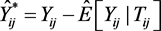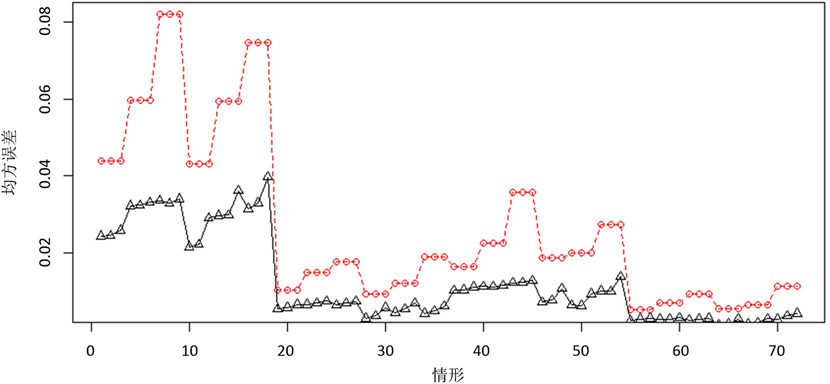﻿ 纵向数据部分线性模型的一种稳健估计方法

# 纵向数据部分线性模型的一种稳健估计方法A Robust Estimation Method for the Partial Linear Models with Longitudinal Data

Abstract: Based on the exponential squared loss function, we propose a robust estimation method for the parametric parts in the partial linear models. By using the kernel approximation and transfor-mation, we first absorb the nonparametric part. Then by using the exponential squared loss func-tion to deduce the influence of outliers, we propose robust generalized estimation equations and empirical likelihood ratio function for estimation and inference. Under some regularity conditions, we show that the resulting estimators are consistent and asymptotic normality. Simulation results also illustrate the robustness and efficiency advantages of our method.

1. 引言

${Y}_{ij}={X}_{ij}^{\text{T}}\beta +g\left({T}_{ij}\right)+{\epsilon }_{ij},\text{\hspace{0.17em}}\text{\hspace{0.17em}}i=1,2,\cdots ,n;\text{\hspace{0.17em}}j=1,2,\cdots ,{m}_{i}$ (1)

Zeger和Diggle  (1994)最早对模型(1)进行了研究，利用后移算法(back-fitting)，结合核估计以及最小二乘估计将参数 $\beta$ 和函数 $g\left(\cdot \right)$ 估计出来。Lin和Carroll  (2001)利用profile估计方程估计参数部分，并结合估计方程与核估计方法估计非参部分。He等  (2002)利用B-样条逼近非参部分，然后选择适当的损失函数用M估计方法估计参数 $\beta$ 以及样条系数。Fan和Li  (2004)利用局部多项式逼近非参部分，在假定工作独立协方差矩阵下，提出两种估计方法：差分估计和profile最小二乘估计。Xue和Zhu  (2008)利用核估计以及差分法消去未知的基准函数 $g\left(\cdot \right)$ ，并利用经验似然(Owen, 1988)  方法估计 $\beta$ 以及进行统计推断。以上方法都没有考虑纵向数据模型中的组内相关性以及估计的稳健性，而在对实际问题的研究中得到的数据常常存在异常值或与假定的分布不符，于是估计的稳健性变得尤为重要。

2. 稳健经验似然的构造

$E\left[{Y}_{ij}|{T}_{ij}\right]=E\left[{X}_{ij}^{\text{T}}|{T}_{ij}\right]\beta +g\left({T}_{ij}\right)$

${Y}_{ij}-E\left[{Y}_{ij}|{T}_{ij}\right]={\left({X}_{ij}-E\left[{X}_{ij}|{T}_{ij}\right]\right)}^{\text{T}}\beta +{\epsilon }_{ij}$

${Y}_{ij}^{*}={Y}_{ij}-E\left[{Y}_{ij}|{T}_{ij}\right]$${X}_{ij}^{*}={X}_{ij}-E\left[{X}_{ij}|{T}_{ij}\right]$ ，则模型变为

${Y}_{ij}^{*}={X}_{ij}^{*\text{T}}\beta +{\epsilon }_{ij},\text{\hspace{0.17em}}\text{\hspace{0.17em}}i=1,2,\cdots ,n;\text{\hspace{0.17em}}j=1,2,\cdots ,{m}_{i}$

${Y}_{i}^{*}={\left({Y}_{i1}^{*},{Y}_{i2}^{*},\cdots ,{Y}_{i{m}_{i}}^{*}\right)}^{\text{T}}$${X}_{i}^{*}={\left({X}_{i1}^{*},{X}_{i2}^{*},\cdots ,{X}_{i{m}_{i}}^{*}\right)}^{\text{T}}$${\epsilon }_{i}={\left({\epsilon }_{i1},{\epsilon }_{i2},\cdots ,{\epsilon }_{i{m}_{i}}\right)}^{\text{T}}$${r}_{i}^{*}\left(\beta \right)={Y}_{i}^{*}-{X}_{i}^{*}\beta$${V}_{i}=Var\left({\epsilon }_{i}\right)={\sigma }_{\epsilon }^{2}{R}_{i}\left(\rho \right)$${R}_{i}\left(\rho \right)$ 为工作相关矩阵， $\rho$ 为未知的参数，注意在随后的矩条件 $E\left[Z\left({\beta }_{0}\right)\right]=0$ 中可将方差 ${\sigma }_{\epsilon }^{2}$ 消去，于是不妨直接假定 ${V}_{i}={R}_{i}\left(\rho \right)$ 。构造辅助随机向量

${Z}_{i}\left(\beta \right)={X}_{i}^{*\text{T}}{V}_{i}^{-1}{h}_{i}^{\gamma }\left({r}_{i}^{*}\left(\beta \right)\right),\text{\hspace{0.17em}}\text{\hspace{0.17em}}i=1,\cdots ,n$

${c}_{ij}=c\left({x}_{ij}\right)=\mathrm{min}\left\{1,{\left\{\frac{{b}_{x}}{{\left({x}_{ij}-{m}_{x}\right)}^{\text{T}}{S}_{x}^{-1}\left({x}_{ij}-{m}_{x}\right)}\right\}}^{\frac{\xi }{2}}\right\}$

$\stackrel{^}{\rho }=\frac{1}{n{H}^{2}}\underset{i=1}{\overset{n}{\sum }}\frac{1}{{m}_{i}\left({m}_{i}-1\right)}\underset{j\ne k}{\sum }{\phi }_{\gamma }\left({e}_{ij}\right){\phi }_{\gamma }\left({e}_{ik}\right)$

$\stackrel{^}{\rho }=\frac{1}{n{H}^{2}}\underset{i=1}{\overset{n}{\sum }}\frac{1}{{m}_{i}-1}\underset{j\le {m}_{i}-1}{\sum }{\phi }_{\gamma }\left({e}_{ij}\right){\phi }_{\gamma }\left({e}_{i,j+1}\right)$

$\stackrel{^}{E}\left[{Y}_{ij}|{T}_{ij}=t\right]=\underset{i=1}{\overset{n}{\sum }}\underset{j=1}{\overset{{m}_{i}}{\sum }}{W}_{ij}\left(t\right){Y}_{ij}$$\stackrel{^}{E}\left[{X}_{ij}|{T}_{ij}=t\right]=\underset{i=1}{\overset{n}{\sum }}\underset{j=1}{\overset{{m}_{i}}{\sum }}{W}_{ij}\left(t\right){X}_{ij}$ ，其中 ${W}_{ij}\left(t\right)={K}_{h}\left({T}_{ij}-t\right)/\underset{k=1}{\overset{n}{\sum }}\underset{l=1}{\overset{{m}_{k}}{\sum }}{K}_{h}\left({T}_{kl}-t\right)$${K}_{h}\left(\cdot \right)=K\left(\cdot /h\right)$$K\left(\cdot \right)$ 是一个核函数。

$L\left(\beta \right)=\mathrm{max}\left\{\underset{i=1}{\overset{n}{\prod }}n{p}_{i}|{p}_{i}\ge 0,\underset{i=1}{\overset{n}{\sum }}{p}_{i}=1,\underset{i=1}{\overset{n}{\sum }}{p}_{i}{\stackrel{^}{Z}}_{i}\left(\beta \right)=0\right\}$

$\underset{i=1}{\overset{n}{\sum }}\frac{{\stackrel{^}{Z}}_{i}\left(\beta \right)}{1+{\lambda }^{\text{T}}{\stackrel{^}{Z}}_{i}\left(\beta \right)}=0$

$l\left(\beta \right)=-\underset{i=1}{\overset{n}{\sum }}\mathrm{log}\left(1+{\lambda }^{\text{T}}{\stackrel{^}{Z}}_{i}\left(\beta \right)\right)$

$\sqrt{n}\left({\stackrel{^}{\beta }}_{MELE}-{\beta }_{0}\right)\stackrel{d}{\to }N\left(0,{\Sigma }_{0}^{-1}{\Omega }_{0}{\Sigma }_{0}^{-1}\right)$

$-2l\left(\beta \right)\stackrel{d}{\to }{\chi }^{2}\left(p\right)$

${I}_{\alpha }=\left\{\beta |-2l\left(\beta \right)\le {\chi }_{1-\alpha }^{2}\left(p\right)\right\}$

3. 算法

Step 1：用核估计计算 $\stackrel{^}{E}\left[{Y}_{ij}|{T}_{ij}\right]$$\stackrel{^}{E}\left[{X}_{ij}|{T}_{ij}\right]$ ，对数据进行变换${\stackrel{^}{X}}_{ij}^{*}={X}_{ij}-\stackrel{^}{E}\left[{X}_{ij}|{T}_{ij}\right]$

Step 2：用GEE估计作为参数估计的初始值 ${\beta }^{\left(0\right)}$ ，令t=1；

Step 3：在估计值为 ${\beta }^{\left(t\right)}$ 时，求出相关性参数的估计 $\stackrel{^}{\rho }$ ，并代入工作相关矩阵得到 ${\stackrel{^}{V}}_{i}={R}_{i}\left(\stackrel{^}{\rho }\right)$ 。通过最小化 $\mathrm{det}\left(Cov\left({\beta }^{\left(t\right)}\right)\right)$ 得到调节参数 $\gamma$ 的估计 ${\gamma }_{opt}^{\left(t\right)}$ ，其中 $\mathrm{det}\left(\cdot \right)$ 表示行列式运算；

$Cov\left({\beta }^{\left(t\right)}\right)={\left[\stackrel{^}{\Sigma }\left({\mu }_{i}\left({\beta }^{\left(t\right)}\right),\stackrel{^}{\rho }\right)\right]}^{-1}\stackrel{^}{\Omega }\left({\mu }_{i}\left({\beta }^{\left(t\right)}\right),\stackrel{^}{\rho }\right){\left[\stackrel{^}{\Sigma }\left({\mu }_{i}\left({\beta }^{\left(t\right)}\right),\stackrel{^}{\rho }\right)\right]}^{-1}$

$\stackrel{^}{\Sigma }\left({\mu }_{i}\left({\beta }^{\left(t\right)}\right),\stackrel{^}{\rho }\right)=\underset{i=1}{\overset{n}{\sum }}{X}_{i}^{\text{T}}{\stackrel{^}{V}}_{i}^{-1}E\left[\frac{\partial {h}_{i}^{\gamma }\left({\mu }_{i}\left({\beta }^{\left(t\right)}\right)\right)}{\partial {\mu }_{i}\left({\beta }^{\left(t\right)}\right)}\right]{X}_{i}$

$\stackrel{^}{\Omega }\left({\mu }_{i}\left({\beta }^{\left(t\right)}\right),\stackrel{^}{\rho }\right)=\underset{i=1}{\overset{n}{\sum }}{X}_{i}^{\text{T}}{\stackrel{^}{V}}_{i}^{-1}\left[{h}_{i}^{\gamma }\left({\mu }_{i}\left({\beta }^{\left(t\right)}\right)\right)\right]{\left[{h}_{i}^{\gamma }\left({\mu }_{i}\left({\beta }^{\left(t\right)}\right)\right)\right]}^{\text{T}}{\stackrel{^}{V}}_{i}^{-1}{X}_{i}$

Step 4：最小化 $-2l\left(\beta ,\stackrel{^}{\rho }\right)$ 得到新的估计值 ${\beta }^{\left(t+1\right)}$

Step 5：令t = t + 1，迭代Step 2~Step 3直到收敛，得到最终的估计值 ${\stackrel{^}{\beta }}_{MELE}$${\stackrel{^}{\gamma }}_{opt}$

4. 模拟

${y}_{ij}={x}_{ij}\beta +g\left({t}_{ij}\right)+{\epsilon }_{ij},\text{\hspace{0.17em}}\text{\hspace{0.17em}}i=1,2,\cdots ,n;\text{\hspace{0.17em}}j=1,2,\cdots ,m$

Table 1. The bias and mean squared error of estimatorsFigure 1. Mean squared error of four estimators under different conditionsTable 2. Coverage probabilities and width of the confidence interval

5. 结论

 Zeger, S.L. and Diggle, P.J. (1994) Semiparametric Models for Longitudinal Data with Application to CD4 Cell Num-bers in HIV Seroconverters. Biometrics, 50, 689.
https://doi.org/10.2307/2532783

 Lin, X. and Carroll, R.J. (2001) Semiparametric Regression for Clustered Data Using Generalized Estimating Equations. Journal of the American Statistical Association, 96, 1045-1056.
https://doi.org/10.1198/016214501753208708

 He, X. and Kim, M.O. (2002) On Marginal Estimation in a Semiparametric Model for Longitudinal Data with Time-Independent Covariates. Metrika, 55, 67-74.
https://doi.org/10.1007/s001840200187

 Fan, J. and Li, R. (2004) New Estimation and Model Selection Procedures for Semiparametric Modeling in Longitudinal Data Analysis. Journal of the American Statistical Association, 99, 710-723.
https://doi.org/10.1198/016214504000001060

 Xue, L.G. and Zhu, L.X. (2008) Empirical Likelihood-Based Inference in a Partially Linear Model for Longitudinal Data. Science in China Series A: Mathematics, 51, 115-130.
https://doi.org/10.1007/s11425-008-0020-4

 Owen, A.B. (1988) Empirical Likelihood Ratio Confidence In-tervals for a Single Functional. Biometrika, 75, 237-249.
https://doi.org/10.1093/biomet/75.2.237

 Liang, K. and Zeger, S.L. (1986) Longitudinal Data Analysis Using Generalized Linear Models. Biometrika, 73, 13-22.
https://doi.org/10.1093/biomet/73.1.13

 Wang, X., Jiang, Y., Huang, M., et al. (2013) Robust Variable Selec-tion with Exponential Squared Loss. Journal of the American Statistical Association, 108, 632-643.
https://doi.org/10.1080/01621459.2013.766613

 He, X., Fung, W.K. and Zhu, Z. (2005) Robust Estimation in Generalized Partial Linear Models for Clustered Data. Journal of the American Statistical Association, 100, 1176-1184.
https://doi.org/10.1198/016214505000000277

Top